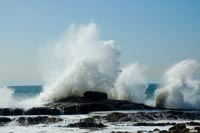# 10.1: Waves

•• Contributed by Miracosta Oceanography 101
• Sourced from Miracosta)

## Waves

This chapter focuses on the phenomena associated waves on bodies of water (oceans, lakes, etc.)
In oceanography, waves are:

• Short-term changes in sea level.
• A wave is energy moving through water.

Waves are generated by a disturbing force - something that transmits energy into a fluid medium (such as wind blowing on water). A pebble hitting a puddle generates a splash that creates ripples (tiny waves) that propagate away from the source (Figure 10.2). The ripples grow smaller as they move away from the splash (source) until they diffuse away with increasing distance, or when it encounters the edge of the puddle.Figure 10.1. A wave crashing onshore releases energy.

Wind is the disturbing force for waves in the ocean and large bodies of water. Waves are also generated by earthquakes, landslides, and volcanic eruptions (producing tsunamis), and tides are produced by gravitational interactions between the Earth, Moon and Sun.Figure 10.2. A splash is an example of a disturbing force creating waves. The ripples radiate away from the splash site, dispersing the energy transmitted to the surface of the water.

### Types of waves

Wind Waves

Tsunamis

Gravity Tides

• Height = range from small ripples up to 60 feet (sometimes higher)
• Speed = 10 – 75 mph
• Periods = 5 – 25 sec.
• Height = open ocean less than 2 feet;
-- onshore up to 300 feet
• Speed = jetliner speeds 400-500 mph
• Wavelength = 100’s of kilometers
• Periods = minutes
Height = up to 50 feet plus
Period 12 ½ to 25 hours

(discussed in Chapter 10)

See: BIGGEST WAVE in the World surfed 100ft at Carlos Burle Portugal (YouTube video)

### Terms Used To Describe Waves

• Crest - the highest part of a passing linear wave
• Trough - the lowest part of a passing linear wave
 - Wavelength (L) = Distance between waves - Period (T) = Time between passing waves - Height (h) = Height from crest to trough (same meaning as amplitude) - Water depth (d) = Average water depth - determines wave behavior

### Characteristics of Waves

Ocean waves are created by wind blowing over water. The distance between two wave crests or two wave troughs is called the wavelength. Wave height is the vertical distance between the highest (crest) and lowest (trough) parts of a wave. (Figure 10.3). Wave period is the time interval between passing wave crests (completing one cycle) and are measured as wave crests pass a stationary point (such as waves passing a buoy or pole on a pier)(Figure 10.4).Figure 10.3. Wavelength and wave height of wave cycles.Figure 10.4. Waves approaching a shoreline arrive at cyclic intervals called a period.